### 当前的情况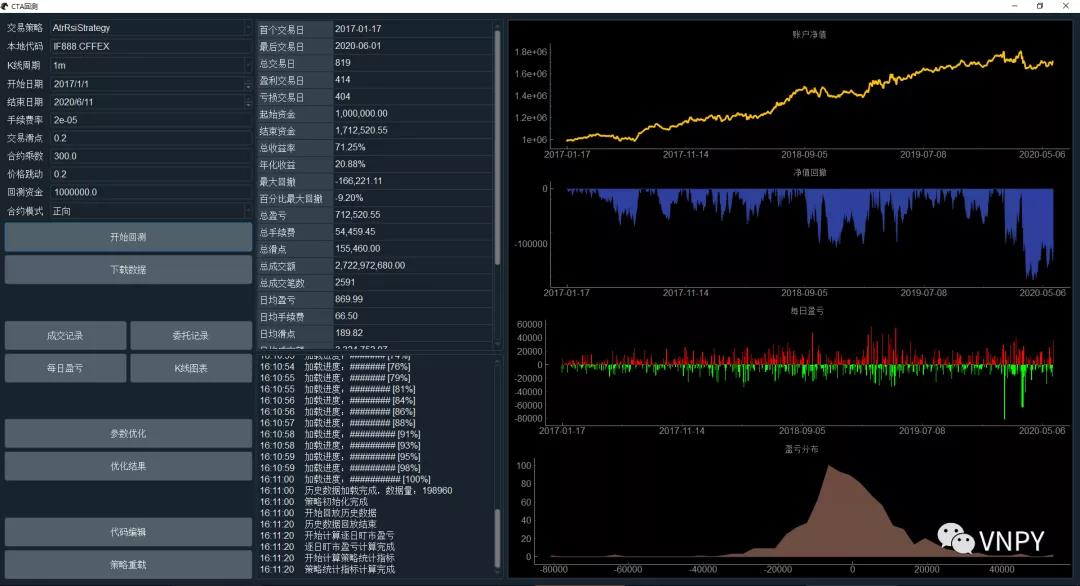CtaStrategy回测引擎作为CtaBacktester图形界面背后的计算引擎，也可以在Jupyter Notebook中直接使用。在CLI命令交互的模式下，用户操作的自由度大大提升，可以实现更加复杂的分析脚本，如【策略组合回测】、【主力切换回测】、【滚动窗口优化】等等：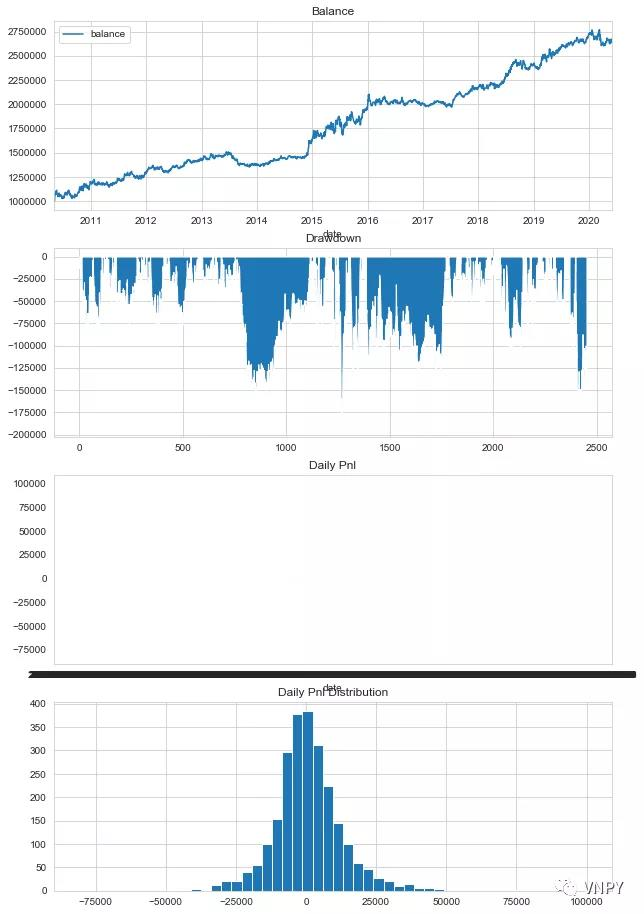### 更好的选择

``pip install plotly``

``````# 加载相关模块
from datetime import datetime

from vnpy.app.cta_strategy.backtesting import BacktestingEngine, OptimizationSetting
from vnpy.app.cta_strategy.strategies.atr_rsi_strategy import AtrRsiStrateg

# 运行策略回测
engine = BacktestingEngine()
engine.set_parameters(
vt_symbol="IF888.CFFEX",
interval="1m",
start=datetime(2010, 1, 1),
end=datetime(2020, 7, 30),
rate=0.3/10000,
slippage=0.2,
size=300,
pricetick=0.2,
capital=1_000_000,
)

# 统计分析结果
engine.run_backtesting()
df = engine.calculate_result()
engine.calculate_statistics()``````

``````import plotly.graph_objects as go
from plotly.subplots import make_subplots``````

``````# 创建一个4行、1列的带子图绘图区域，并分别给子图加上标题
fig = make_subplots(rows=4, cols=1, subplot_titles=["Balance", "Drawdown", "Daily Pnl", "Pnl Distribution"], vertical_spacing=0.06)

# 第一张：账户净值子图，用折线图来绘制

# 第二张：最大回撤子图，用面积图来绘制
fig.add_trace(go.Scatter(x=df.index, y=df["drawdown"], fillcolor="red", fill='tozeroy', line={"width": 0.5, "color": "red"}, name="Drawdown"), row=2, col=1)

# 第三张：每日盈亏子图，用柱状图来绘制

# 第四张：盈亏分布子图，用直方图来绘制

# 把图表放大些，默认小了点
fig.update_layout(height=1000, width=1000)

# 将绘制完的图表，正式显示出来
fig.show()``````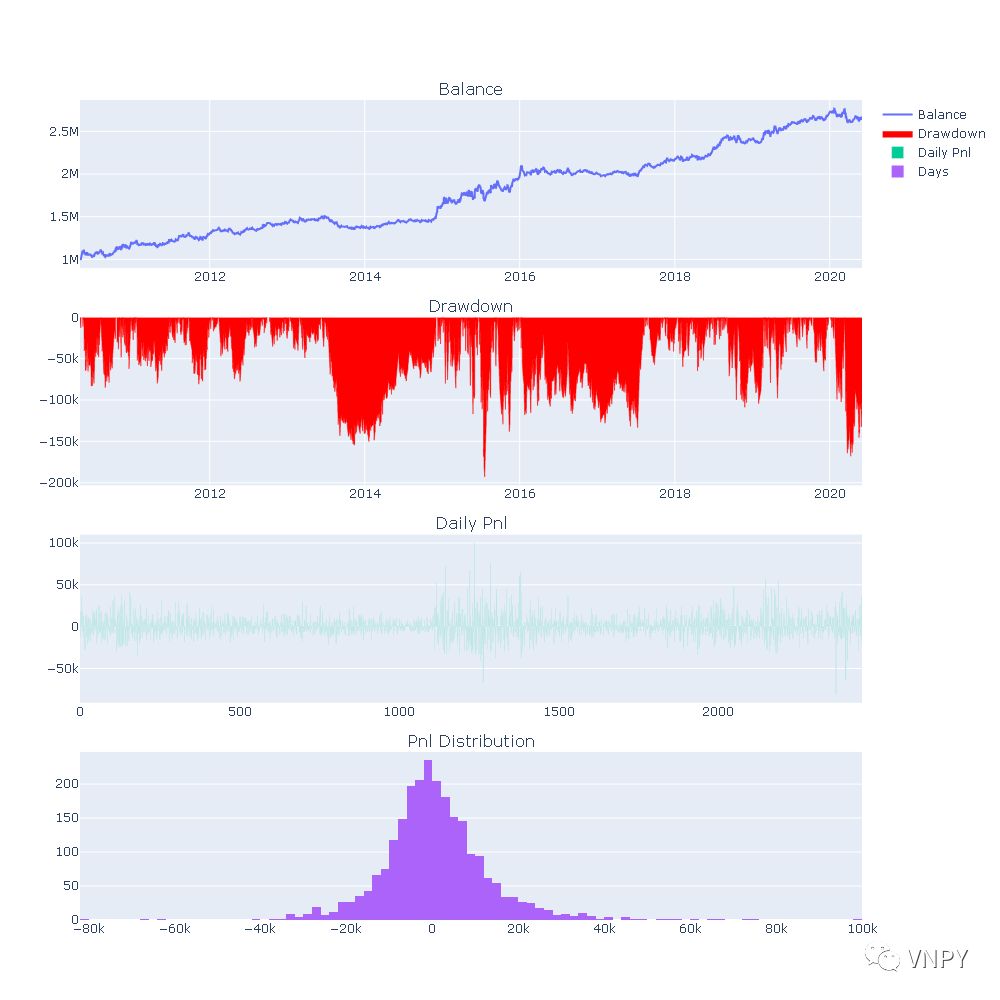### 3D参数曲面

``````# 创建优化配置
setting = OptimizationSetting()

# 选择夏普比率作为目标函数
setting.set_target("sharpe_ratio")

# 选择优化rsi_length和atr_length两个参数

# 通过多进程穷举算法来执行优化
result = engine.run_optimization(setting)``````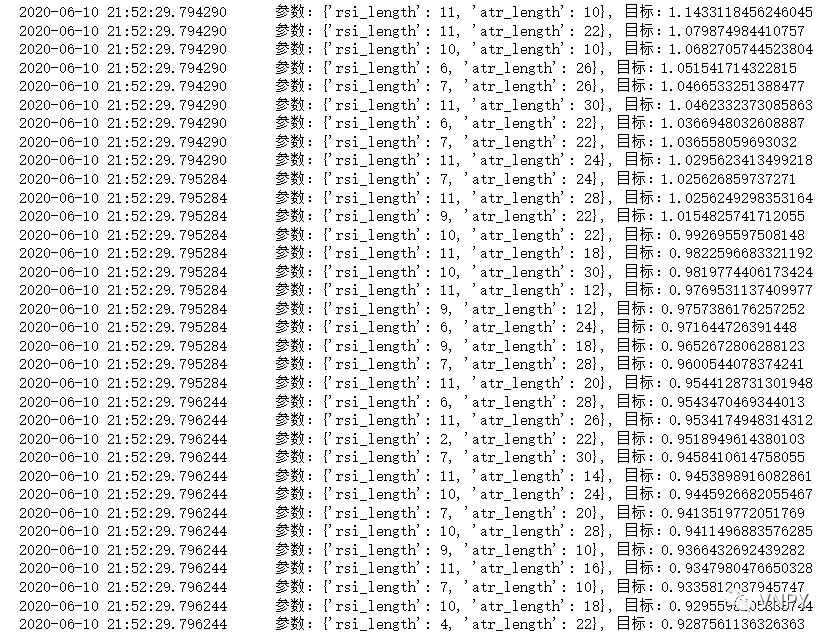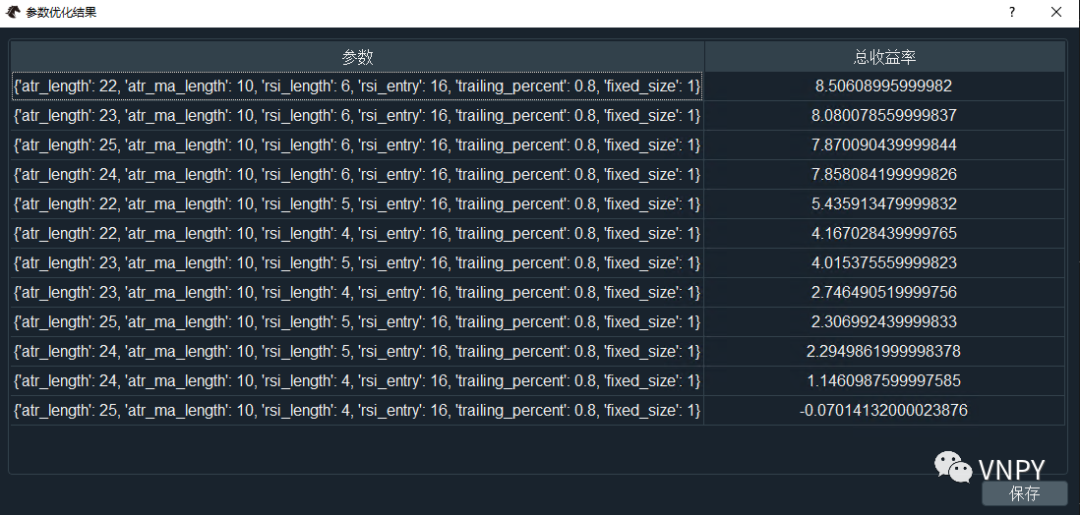``````# 直接取出X、Y轴
x = setting.params["atr_length"]
y = setting.params["rsi_length"]

# 通过映射的方式取出Z轴
z_dict = {}
for param_str, target, statistics in result:
param = eval(param_str)
z_dict[(param["atr_length"], param["rsi_length"])] = target

z = []
for x_value in x:
z_buf = []
for y_value in y:
z_value = z_dict[(x_value, y_value)]
z_buf.append(z_value)
z.append(z_buf)``````

``````fig = go.Figure(data=[go.Surface(z=z, x=x, y=y)])
fig.update_layout(
title='优化结果', autosize=False,
width=600, height=600,
scene={
"xaxis": {"title": "atr_length"},
"yaxis": {"title": "rsi_length"},
"zaxis": {"title": setting.target_name},
},
margin={"l": 65, "r": 50, "b": 65, "t": 90}
)
fig.show()``````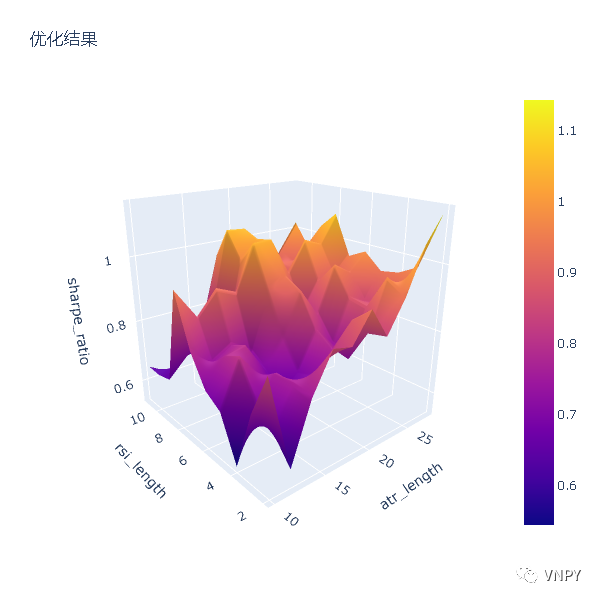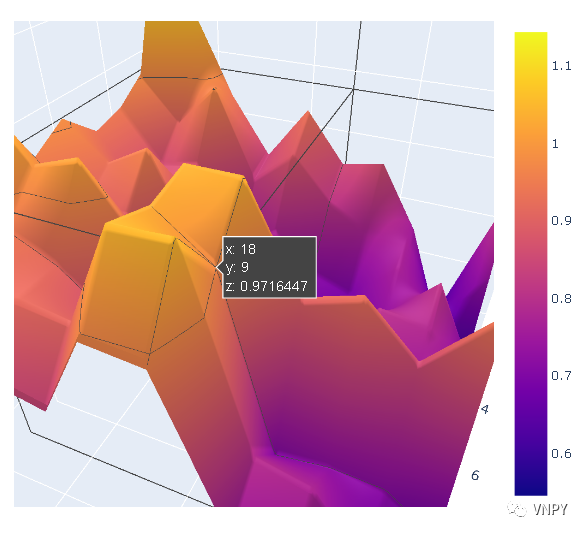### 涨价通知

【vn.py全实战进阶 - CTA策略】课程销量已经达到778份。